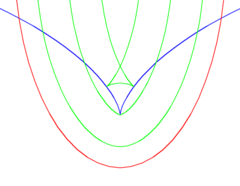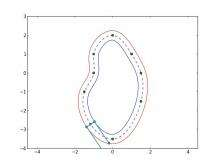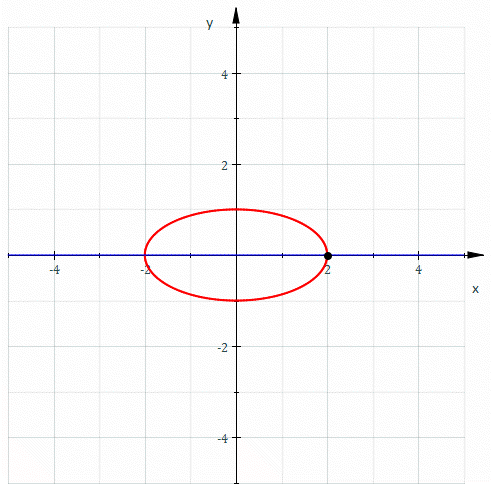# Parallel curve

Not to be confused with parallel transport.An ellipse (red), its evolute (blue) and some parallel curves (green). Note how the parallel curves have cusps when they just touch (rather than intersect) the evolute at two distinct points.Inner and outer parallel curves to a parametric cubic spline loop.Animation of the construction of the parallel curves : ellipse (red), evolute (astroid, blue) and animation of parallel curves in black.

A parallel of a curve is the envelope of a family of congruent circles centered on the curve. It generalises the concept of parallel lines. It can also be defined as a curve whose points are at a fixed normal distance from a given curve. These two definitions are not entirely equivalent as the latter assumes smoothness, whereas the former does not.

In Computer-aided design the preferred term for a parallel curve is offset curve  (In other geometric contexts, the term offset can also refer also to translation.) Offset curves are important for example in numerically controlled machining, where they describe for example the shape of the cut made by a round cutting tool of a two-axis machine. The shape of the cut is offset from the trajectory of the cutter by a constant distance in the direction normal to the cutter trajectory at every point.

In the area of 2D computer graphics known as vector graphics, the (approximate) computation of parallel curves is involved in one of the fundamental drawing operations, called stroking, which is typically applied to polylines or polybeziers (themselves called paths) in that field.

Except in the case of a line or circle, the parallel curves have a more complicated mathematical structure than the progenitor curve. For example, even if the progenitor curve is smooth, its offsets may not be so; this property is illustrated in the adjacent figure using a parabola as progenitor curve. In general, even if a curve is rational, its offsets may not be so. For example, the offsets of a parabola are rational curves, but the offsets of an ellipse or of a hyperbola are not rational, even though these progenitor curves themselves are rational.

The notion also generalizes to 3D surfaces, where it is called offset surface. Increasing a solid volume by a (constant) distance offset is sometimes called dilation. The opposite operation is sometimes called shelling.

## Alternative definitions

Alternatively, one can fix a circle and a point on the curve and take the envelope of the translations taking that point to the circle.

Tracing the center of a circle rolled along the curve (see roulette) would give one branch of a parallel.

## Parametric curve

For a parametrically defined curve, the following equations define one branch of its parallel curve with distance(the other branch is obtained with):## Examples

Some examples:

## Geometric properties

As for parallel lines, a normal line to a curve is also normal to its parallels.

When parallel curves are constructed they will have cusps when the distance from the curve matches the radius of curvature. These are the points where the curve touches the evolute.

If the progenitor curve is a boundary of a planar set and its parallel curve is without self-intersections, then the latter is the boundary of the Minkowski sum of the planar set and the disk of the given radius.

## Algorithms

An efficient algorithm for offsetting is the level approach described by Kimel and Bruckstein (1993). 

There are numerous approximation algorithms for this problem. For a 1997 survey see.

## Generalizations

The problem generalizes fairly obviously to higher dimensions e.g. to offset surfaces, and slightly less trivially to pipe surfaces. Note that the terminology for the higher-dimensional versions varies even more widely than in the planar case, e.g. other authors speak of parallel fibers, ribbons, and tubes. For curves embedded in 3D surfaces the offset may be taken along a geodesic.

Another way to generalize it is (even in 2D) to consider a variable distance, e.g. parametrized by another curve. One can for example stroke (envelope) with an ellipse instead of circle as it is possible for example in METAFONT. More recently Adobe Illustrator has added somewhat similar facility in version CS5, although the control points for the variable width are visually specified. In contexts where it's important to distinguish between constant and variable distance offsetting the acronyms CDO and VDO are sometimes used.

## References

1. Willson, Frederick Newton (1898). Theoretical and Practical Graphics. Macmillan. p. 66. ISBN 1-113-74312-3.
2. Satyan L. Devadoss; Joseph O'Rourke (2011). Discrete and Computational Geometry. Princeton University Press. pp. 128–129. ISBN 1-4008-3898-3.
3. J. Rafael Sendra; Franz Winkler; Sonia Pérez-Diaz (2007). Rational Algebraic Curves: A Computer Algebra Approach. Springer Science & Business Media. p. 10. ISBN 978-3-540-73724-7.
4. Max K. Agoston (2005). Computer Graphics and Geometric Modelling: Mathematics. Springer Science & Business Media. p. 586. ISBN 978-1-85233-817-6.
5. John Vince (2006). Geometry for Computer Graphics: Formulae, Examples and Proofs. Springer Science & Business Media. p. 293. ISBN 978-1-84628-116-7.
6. Duncan Marsh (2006). Applied Geometry for Computer Graphics and CAD (2nd ed.). Springer Science & Business Media. p. 107. ISBN 978-1-84628-109-9.
7. Max K. Agoston (2005). Computer Graphics and Geometric Modelling. Springer Science & Business Media. pp. 638–645. ISBN 978-1-85233-818-3.
8. http://faculty.engineering.ucdavis.edu/farouki/wp-content/uploads/sites/41/2013/02/Introduction-to-PH-curves.pdf, p. 16 "taxonomy of offset curves"
9. Kimmel and Bruckstein (1993) Shape offsets via level sets CAD (Computer Aided Design) 25(3):154162.
10. http://www.computer.org/csdl/mags/cg/1997/03/mcg1997030062.pdf
11. Helmut Pottmann; Johannes Wallner (2001). Computational Line Geometry. Springer Science & Business Media. pp. 303–304. ISBN 978-3-540-42058-3.
12. Gregory S. Chirikjian (2009). Stochastic Models, Information Theory, and Lie Groups, Volume 1: Classical Results and Geometric Methods. Springer Science & Business Media. pp. 171–175. ISBN 978-0-8176-4803-9.
13. Muhammad Sarfraz, ed. (2003). Advances in geometric modeling. Wiley. p. 72. ISBN 978-0-470-85937-7.
14. Eric L. Brechner (1992). "5. General Offset Curves and Surfaces". In Robert E. Barnhill. Geometry Processing for Design and Manufacturing. SIAM. pp. 101–. ISBN 978-0-89871-280-3.
15. "Properties of Generalized Offset Curves and Surfaces". Hindawi.com. Retrieved 2014-08-15.
16. https://www.tug.org/TUGboat/tb16-3/tb48kinc.pdf
17. http://design.tutsplus.com/tutorials/illustrator-cs5-variable-width-stroke-tool-perfect-for-making-tribal-designs--vector-4346 application of the generalized version in Adobe Illustrator CS5 (also video)